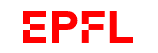• Mesh-adaptive numerical methods in various applied problems
• A posteriori error control and verification of numerical solutions
• Validation of mathematical models used in computer simulation
• Nonlinear computational partial differential equations
• Novel discretization methods for the numerical approximation of PDEs21 June 2022 — 23 June 2022
2:40 pm

SwissTech Convention Center Lausanne